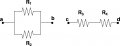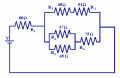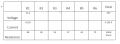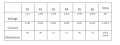# Help on Combination circuit

#### expertz

Joined Oct 20, 2022
9
Hi, I am in Grade 11 and I have been struggling with this circuit, and these kinds of circuits that splits at the large box into different resistors are the only ones that make me confused. How would I find the current and voltage of R2, R3, R4, R5, and R6? I already know there's 14.16V and 0.073A going in the box but that's all I know for now because of R1's voltage drop. What can I do next? I have a test tomorrow and these types of circuits will be on there. Thanks!

#### dl324

Joined Mar 30, 2015
15,486
Welcome to AAC!
What can I do next?
Do you know how to work with current dividers?

For schoolwork, you have to do most of the work because you'll learn more that way. We can only offer guidance.

#### MrChips

Joined Oct 2, 2009
27,726
Learn how to work with resistors in series and resistors in parallel.Add resistors in series: Reff = R3 + R4
For resistors in parallel, add the reciprocals: 1/Reff = 1/R1 + 1/R2In the circuit above,
Which two resistors do you see are in parallel?
Which two resistors do you see are in series?

#### crutschow

Joined Mar 14, 2008
31,151
Do you know how to calculate resistance of two parallel resistors so they can be replaced by one equivalent resistance?

#### dl324

Joined Mar 30, 2015
15,486
BTW, I disagree with the current shown for R1. I did the arithmetic several times to make sure...

#### crutschow

Joined Mar 14, 2008
31,151
BTW, I disagree with the current shown for R1. I did the arithmetic several times to make sure...
I also calculated a different value, which agrees with my simulation of the circuit.
It's above 100mA with 14.16V applied to the network.

#### expertz

Joined Oct 20, 2022
9
Learn how to work with resistors in series and resistors in parallel.

View attachment 278953

Add resistors in series: Reff = R3 + R4
For resistors in parallel, add the reciprocals: 1/Reff = 1/R1 + 1/R2

View attachment 278954

In the circuit above,
Which two resistors do you see are in parallel?
Which two resistors do you see are in series?
I know how they work, however I dont know how the current splits at that junction. If I made an equivalent resistance by combining the resistors, it wouldn’t show the correct currents and voltages for each individual resistor, but the entire equivalent resistance of multiple resistors.

I’ve tried to use Ohms law by using 0.073A for each resistor but the current was always higher than my total current

#### expertz

Joined Oct 20, 2022
9
BTW, I disagree with the current shown for R1. I did the arithmetic several times to make sure...
How would it be different values? I did I=V/R (20/275.3) and I ended up at 0.073A. It runs across R1 yes?

#### expertz

Joined Oct 20, 2022
9
Do you know how to calculate resistance of two parallel resistors so they can be replaced by one equivalent resistance?
If I made an equivalent resistance by combining the resistors, it wouldn’t show the correct currents and voltages for each individual resistor, but the entire equivalent resistance of multiple resistors. Or, I’m just doing it wrong.

#### WBahn

Joined Mar 31, 2012
27,940
Hi, I am in Grade 11 and I have been struggling with this circuit, and these kinds of circuits that splits at the large box into different resistors are the only ones that make me confused. How would I find the current and voltage of R2, R3, R4, R5, and R6? I already know there's 14.16V and 0.073A going in the box but that's all I know for now because of R1's voltage drop. What can I do next? I have a test tomorrow and these types of circuits will be on there. Thanks!
You talk about some "box" but then don't define what it is. Instead, you leave it the reader to figure it out. Don't do that. It just leads to miscommunication and frustration on everyone's part. I'm inferring that your "box" consists of all of the resistors except R1?

When you say you already know 14.16 V (which does not go "into" the box, but rather is the voltage appearing "across" the box) and 73 mA, is that because that is what you calculated, or because that was part of the given information?

What were you actually given? I'm thinking that you were given the values of all of the resistors and all of the values in the "Total" column. Is that correct?

Let's put some bounds on what the largest and smallest values the total resistance can be.

Hopefully one thing you have learned is that when you put two resistors in series, the combined resistance is always larger than either of the two resistors. We can see that the total resistance in the circuit consists of R1 in series with something (the "box"), so that means that our total resistance can't be any smaller than 80 Ω.

So we have

Rtot > 80 Ω

What about bounds on the resistance of the "box"?

Hopefully it's been pointed out that when you put two resistors in parallel, that the combined resistance is less than either of the two resistors. Within the box, we see that we have the series combination of R2 and R3 and this combination is in parallel with some combination of the other three resistors. So we know that

Rbox < (the series combination of R2 and R3).

So the MAX that the total resistance can possibly be is the series combination of 80 Ω and whatever the series combination of R2 and R3 is.

Given that, can you put a number to the maximum value of Rtot?

How does that compare with the Rtot in your table?

#### WBahn

Joined Mar 31, 2012
27,940
I know how they work, however I dont know how the current splits at that junction. If I made an equivalent resistance by combining the resistors, it wouldn’t show the correct currents and voltages for each individual resistor, but the entire equivalent resistance of multiple resistors.

I’ve tried to use Ohms law by using 0.073A for each resistor but the current was always higher than my total current
The idea is to combine them in order to tease more information out of the circuit and then uncombine them in order to apply that newfound information.

Remember that Ohms Law relates the resistance of a specific resistor to the voltage across THAT resistor and current through THAT resistor.

#### WBahn

Joined Mar 31, 2012
27,940
How would it be different values? I did I=V/R (20/275.3) and I ended up at 0.073A. It runs across R1 yes?
So where did the 20 V and the 275.3 Ω values come from?

Were they given?

Are they reasonable, given the values of the resistances in the circuit?

Can you combine all six resistors into a single equivalent resistance?

Does that value match 275.3 Ω?

#### WBahn

Joined Mar 31, 2012
27,940
If I made an equivalent resistance by combining the resistors, it wouldn’t show the correct currents and voltages for each individual resistor, but the entire equivalent resistance of multiple resistors. Or, I’m just doing it wrong.
Yes. it will give you the total resistance, which will let you find the total current if you know the voltage (the 20 V) or find the total voltage if you know the total current (the 73 mA). It will let you compare these to the given values to ascertain whether the given values make sense?

#### expertz

Joined Oct 20, 2022
9
So where did the 20 V and the 275.3 Ω values come from?

Were they given?

Are they reasonable, given the values of the resistances in the circuit?

Can you combine all six resistors into a single equivalent resistance?

Does that value match 275.3 Ω?
OH never mind about the incorrect resistance. I had 128.8 Ohms (which I think is correct) but I tried a different voltage and I got something difference and did not remove its
So where did the 20 V and the 275.3 Ω values come from?

Were they given?

Are they reasonable, given the values of the resistances in the circuit?

Can you combine all six resistors into a single equivalent resistance?

Does that value match 275.3 Ω?
OH, never mind. I had 128.8 ohms as the total resistance before I changed the voltage and forgot to remove that value. I’ll let you know the part I got stuck after I attempt this again

#### MrChips

Joined Oct 2, 2009
27,726
I know how they work, however I dont know how the current splits at that junction. If I made an equivalent resistance by combining the resistors, it wouldn’t show the correct currents and voltages for each individual resistor, but the entire equivalent resistance of multiple resistors.

I’ve tried to use Ohms law by using 0.073A for each resistor but the current was always higher than my total current
If you know how to work with resistors in series and parallel then do it and show us how you do it.
This has to be the first step in order to calculate the current flowing in the circuit.
Do this and then we will move on to the next step.

#### expertz

Joined Oct 20, 2022
9
If you know how to work with resistors in series and parallel then do it and show us how you do it.
This has to be the first step in order to calculate the current flowing in the circuit.
Do this and then we will move on to the next step.This is the updated table I have now. All I need now is a way to calculate the current or voltage of R2, R3, R4, R5, and R6. I don't know where to start here. I think there's 7.6 V and 0.155 A going through the "box" of resistors.

#### WBahn

Joined Mar 31, 2012
27,940
So if there's 7.6 V across the box, what is the voltage across the combined resistances of R2 and R3?

#### WBahn

Joined Mar 31, 2012
27,940
We all make mistakes in our math, so we need to develop ways to sanity check our work in order to avoid going down rabbit holes and waste precious time doing work that is guaranteed to be useless.

A big part of this is tracking units properly at every step of the work. Another is learning to quickly estimate bounds on what the answer (including key intermediate answers) can be. If we get a result outside those bounds, we know we have done something wrong, either with the answer or with the bounds, and should stop and resolve it before moving forward.

In this circuit, a quick visual glance should tell you that the total resistance can be no more than 180 Ω. So when you got 275.3 Ω, huge warning bells should have started sounding.

Similarly, you know the total resistance has to be at least 80 Ω, so if you had gotten a total of 48 Ω (due to forgetting to add in the 80 Ω after finding the resistance of the "box", which would be an easy mistake to make), those same warning bells should have gone off.

We can actually narrow the range of Rtot down a lot more without actually calculating any of the combined resistances.

BOUNDS ON SERIES RESISTORS

Given two resistors, Ra and Rb such that Ra <= Rb:

The combined resistance MUST be larger than the larger resistor, no matter what the other resistor is.

Furthermore, if must also be larger than twice the smaller resistor.

So we have two limits on the lower bound and the actual lower bound is the larger of these (since both have to be satisfied).

Looking at the other bound, the MOST that the combined resistance can be is twice the value of the larger resistor.

max(2·Ra, Rb) <= Rab <= 2·Rb

BOUNDS ON PARALLEL RESISTORS

Given two resistors, Ra and Rb such that Ra <= Rb:

The combined resistance MUST be less than the smaller resistor, no matter what the other resistor is.

Furthermore, it must be less than half the value of the larger resistor.

So we have two limits on the upper bound and the actual upper bound is the smaller of these (since both have to be satisfied).

Looking at the other bound, the LEAST that the combined resistance can be is half of the smaller resistor.

Ra/2 <= Rab <= min(Ra, Rb/2)

RIGOROUS BOUNDS ON Rtot

So, with this in mind, let's calculate some bounds on Rtot for your circuit.

Looking first at R4 and R5, let's call the combined resistance R45.

Ra/2 <= Rab <= min(Ra, Rb/2)
37 Ω/2 <= R45 <= min(37 Ω, 45 Ω/2)
18.5 Ω <= R45 <= min(37 Ω, 22.5 Ω)
18 Ω <= R45 <= 23 (expanded the range to avoid fractional resistances)

Adding in R6 in series, we have

max(2·Ra, Rb) <= Rab <= 2·Rb
max(2·23 Ω, 75 Ω) <= R456 <= 2·75 Ω
75 Ω <= R456 <= 150 Ω

In practice, we would just add the 75 Ω to our range of values for R45 and get limits of 93 Ω and 98 Ω, but I said I was going to work this one without actually doing any combining, but well come back to this later.

For R2 and R3, we get

98 Ω <= R23 <= 102 Ω/2

Again, in practice, we would just add this together by inspection and get R23 = 100 Ω.

Now it's time to combine R23 and R456 in parallel to get bounds on the box.

Ra/2 <= Rab <= min(Ra, Rb/2)

98 Ω <= R23 <= 102 Ω/2
75 Ω <= R456 <= 150 Ω

Here the bounds for R23 and R456 overlap, so which is Ra and which is Rb. The simple way, even though it produces looser bounds, is just to use the extreme values from both ranges.

75 Ω/2 <= Rbox <= min(75 Ω, 150 Ω/2)
37.5 Ω <= Rbox <= 75 Ω

So now we can get bounds on Rtot, which is the series combination of R1 and Rbox.

max(2·Ra, Rb) <= Rab <= 2·Rb

R1 = 80 Ω
37.5 Ω <= Rbox <= 75 Ω

max(2·37.5 Ω, 80 Ω) <= Rtot <= 2·80 Ω

80 Ω <= Rtot <= 160 Ω

This isn't much tighter than our quick one, which put the upper limit at 180 Ω.

QUICK BOUNDS ON Rtot

The above was done the long way in order to establish the idea, but we can get good results quickly but applying these concepts doing nothing but mental arithmetic.

First, let's find an upper bound.

R45 can be no more than the smaller of R4 and R5, so let's call it 37 Ω. But it also can't be any larger than half of 45 Ω, so we change that to 23 Ω

Adding R6 to that we get 98 Ω.

That is in parallel with (R2+R3)=100 Ω, These are basically the same and the resistance has to be less than 50 Ω.

That makes Rtot <= 130 Ω

Now for the lower bound.

R45 has to be at least half of 37 Ω, call that 18 Ω.

Putting this in parallel with 100 Ω gives us something that can be no smaller than 46 Ω, which in turn means that Rtot >= 126 Ω.

We we were able to very quickly establish that

126 Ω <= Rtot <= 130 Ω

In many instances, this alone is good enough to simply take the average of 128 Ω and call it a day since that puts the actual value within 2% and we are probably working with resistors that have worse tolerances than that.

#### expertz

Joined Oct 20, 2022
9
So if there's 7.6 V across the box, what is the voltage across the combined resistances of R2 and R3?
I don't know how to get there, because what I tried was combine 49 + 51 which is 100. Then I used I = V/R (7.6/100) to get 0.076 which is the current at the top part of the "box". I couldn't really do V= IR because that didn't work for me.

Instead I did 0.155 (total current) - 0.076 to get 0.079 A on the other branch, then I applied 0.079 A on the lone R6 then found the voltage (5.925 V) from there. I then did 7.6 - 5.925 which was 1.675 V, which I used to solve that side. I noticed the bottom part used 5.925 so then I did 7.6 - 5.925 and I got 1.675 V AGAIN, then I used it to solve the top side.

I don't know if it's correct since R2 and R3's current did not add to 0.076 A - if not can you explain what I could do?